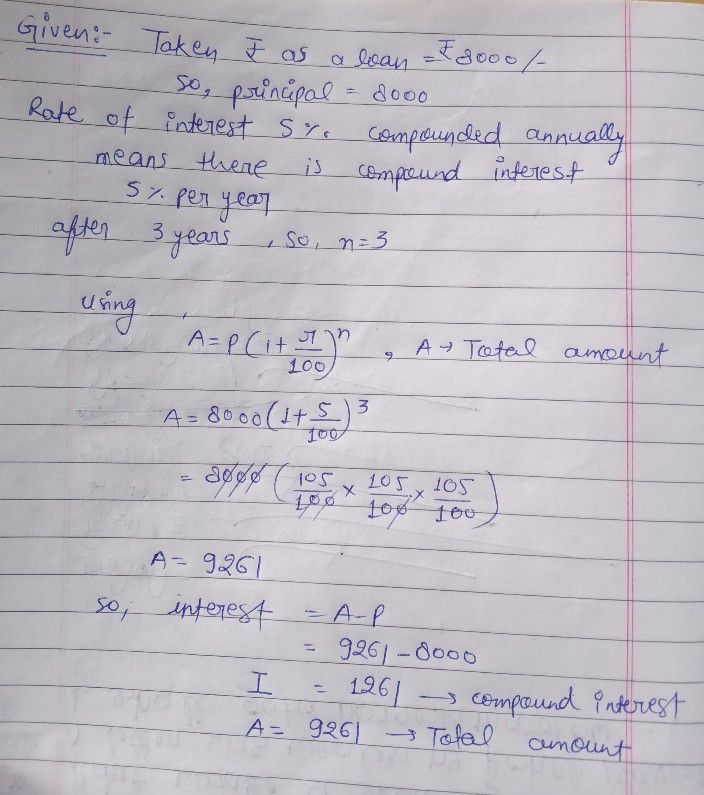Symbol
Problem$7.$ To renovate his shop, Anurag obtained $a$ $loa$ loan of $z8000$ from $a$ bank. If the rate of interest at $50$ per annum is compounded annually, calculate the $C$ compound interest that Anurag will have to pay after $3$ years.
Other
SolutionStudent
thanks
sir